# Thread: stuck on these two integrals

1. ## stuck on these two integrals

Evaluate..
a)and..

b)I'm stuck on these two..can't get the answer correctly..

2.Originally Posted by Jgirl689Evaluate..
a)and..

b)I'm stuck on these two..can't get the answer correctly..
'Correctly' ? Please show what you did...

Anyway, these are both standard u-substitutions - where, to get the anti-derivative, you spot that the integrand is a product, one part of which is uncannilly like the derivative of the other. Or, more precisely, is nearly the derivative of some 'inner' part of the other part.

So, for a), your u is going to be the denominator, and for b) u is the interior of the square root.

Edit:

Just in case a picture helps...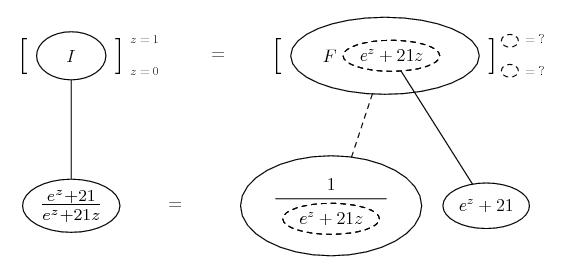... where... is the chain rule. Straight continuous lines differentiate downwards (integrate up) with respect to x, and the straight dashed line similarly but with respect to the dashed balloon expression (the inner function of the composite which is subject to the chain rule).

The general drift...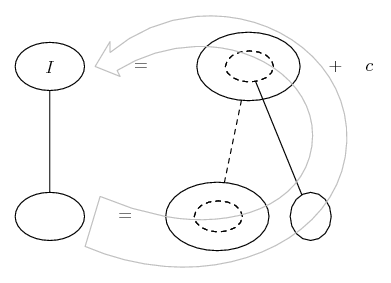And the rest...

Spoiler: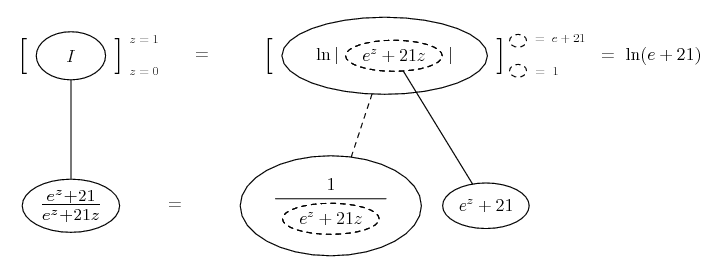Then, for b...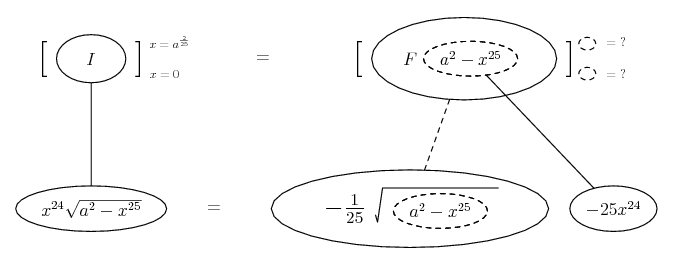Spoiler: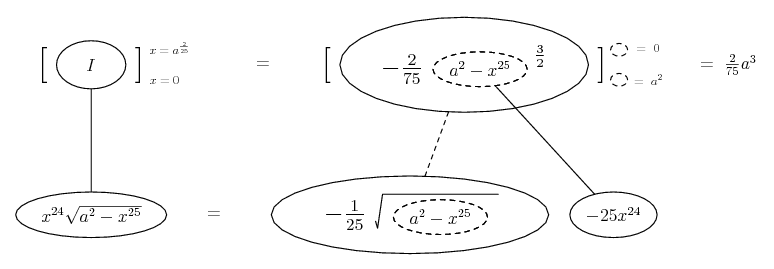________________________________________

Don't integrate - balloontegrate!

Balloon Calculus; standard integrals, derivatives and methods

Balloon Calculus Drawing with LaTeX and Asymptote!

3.Originally Posted by Jgirl689Evaluate..
a)and..

b)I'm stuck on these two..can't get the answer correctly..
Notice that:
$\displaystyle (e^z+21z)'=e^z+21$

So the integral is of the form $\displaystyle \int\frac{f'(x)}{f(x)}dx=ln(f(x))+C$

$\displaystyle \int_0^1\frac{e^z+21}{e^z+21z}dz=ln(e^z+21z)]_0^1$

4. notice that the derivative of a^2 - x^25 is -25x^24 and notice that you have an x^24 sitting outside the radical. if the derivative of a function can be found in the integrand, its a good indication of u-substitution.

#### Search Tags

integrals, stuck